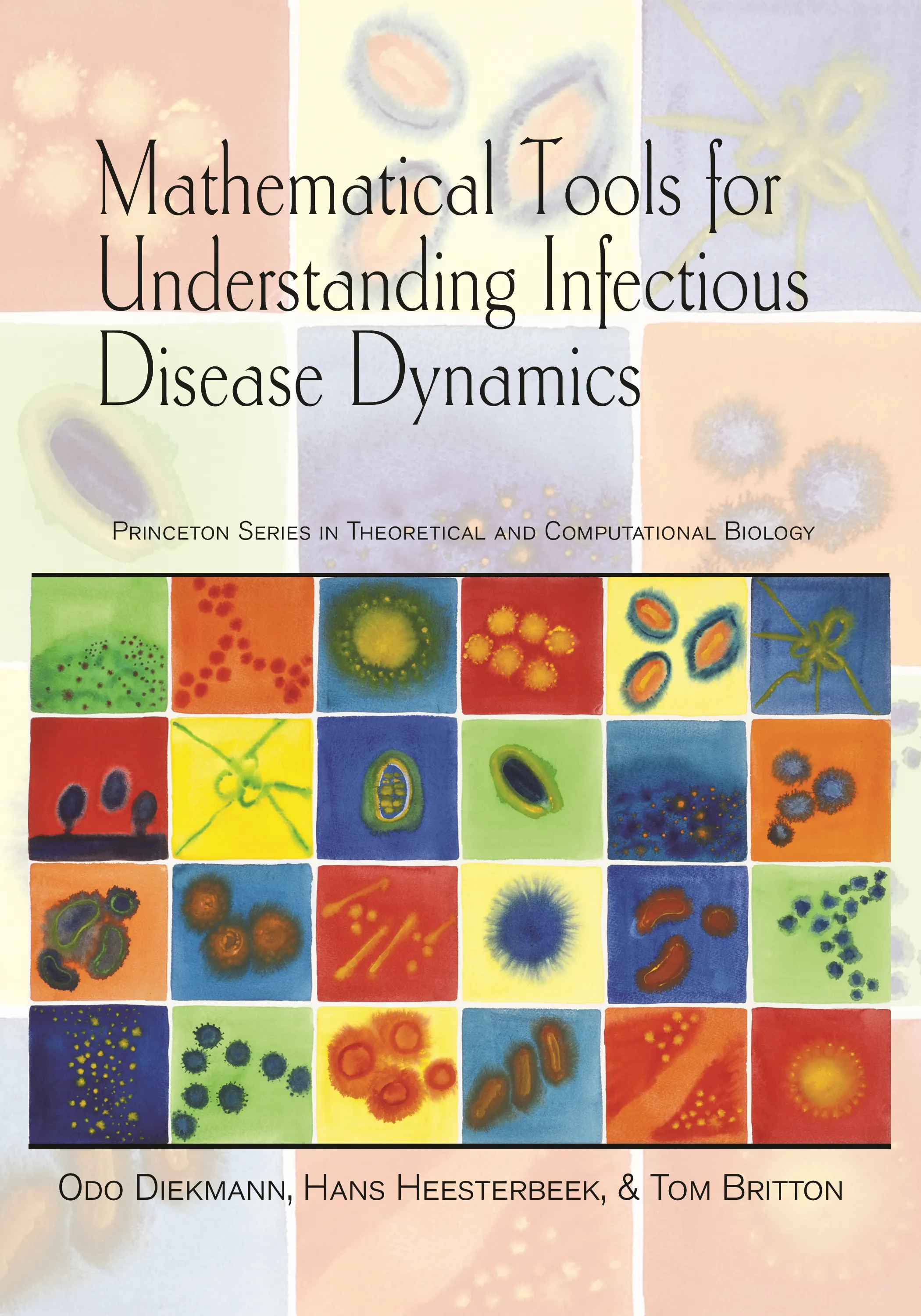Mathematical Tools for Understanding Infectious Disease Dynamics

Odo Diekmann

ebooks

Many of our ebooks are available for purchase from these online vendors:

Many of our ebooks are available through library electronic resources including these platforms:

Mathematical Tools for Understanding Infectious Disease Dynamicsebook

ISBN:
Published:
Nov 18, 2012
2013
Illus:
53 line illus. 1 table.

Mathematical modeling is critical to our understanding of how infectious diseases spread at the individual and population levels. This book gives readers the necessary skills to correctly formulate and analyze mathematical models in infectious disease epidemiology, and is the first treatment of the subject to integrate deterministic and stochastic models and methods.

Mathematical Tools for Understanding Infectious Disease Dynamics fully explains how to translate biological assumptions into mathematics to construct useful and consistent models, and how to use the biological interpretation and mathematical reasoning to analyze these models. It shows how to relate models to data through statistical inference, and how to gain important insights into infectious disease dynamics by translating mathematical results back to biology. This comprehensive and accessible book also features numerous detailed exercises throughout; full elaborations to all exercises are provided.

• Covers the latest research in mathematical modeling of infectious disease epidemiology
• Integrates deterministic and stochastic approaches
• Teaches skills in model construction, analysis, inference, and interpretation
• Features numerous exercises and their detailed elaborations
• Motivated by real-world applications throughout